# Test: Delta Star & Star Delta

## 20 Questions MCQ Test Network Theory (Electric Circuits) | Test: Delta Star & Star Delta

Description
Attempt Test: Delta Star & Star Delta | 20 questions in 20 minutes | Mock test for Electrical Engineering (EE) preparation | Free important questions MCQ to study Network Theory (Electric Circuits) for Electrical Engineering (EE) Exam | Download free PDF with solutions
QUESTION: 1

### The value of the 3 resistances when connected in star connection is_________​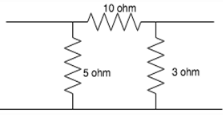Solution:

Following the delta to star conversion:
R1=10*5/(10+5+3)
R2=10*3/(10+5+3)
R3=5*3/(10+5+3).

QUESTION: 2

### Which, among the following is the right expression for converting from delta to star?

Solution:

After converting to star, each star connected resistance is equal to the product of the resistances it is connected to and the total sum of the resistances. Hence R1=Ra*Rb/(Ra+Rb+Rc), R2=Rb*Rc/(Ra+Rb+Rc), R3=Rc*Ra/(Ra+Rb+Rc).

QUESTION: 3

### Find the equivalent star network.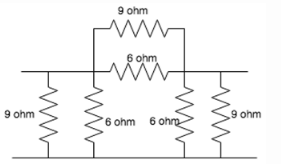Solution:

The 6 ohm and 9 ohm resistances are connected in parallel. Their equivalent resistances are: 6*9/(9+6)=3.6 ohm.
The 3 3.6 ohm resistors are connected in delta. Converting to star:
R1=R2=R3= 3.6*3.6/(3.6+3.6+3.6)=1.2 ohm.

QUESTION: 4

Star connection is also known as__________

Solution:

The star connection is also known as the Y-connection because its formation is like the letter Y.

QUESTION: 5

Rab is the resistance between the terminals A and B, Rbc between B and C and Rca between C and A. These 3 resistors are connected in delta connection. After transforming to star, the resistance at A will be?

Solution:

When converting from delta to star, the resistances in star connection is equal to the product of the resistances it is connected to, divided by the total sum of the resistance.
Hence Rab*Rac/(Rab+Rbc+Rca).

QUESTION: 6

Rab is the resistance between the terminals A and B, Rbc between B and C and Rca between C and A. These 3 resistors are connected in delta connection. After transforming to star, the resistance at B will be?

Solution:

When converting from delta to star, the resistances in star connection is equal to the product of the resistances it is connected to, divided by the total sum of the resistance.
Hence Rab*Rbc/(Rab+Rbc+Rca).

QUESTION: 7

Rab is the resistance between the terminals A and B, Rbc between B and C and Rca between C and A. These 3 resistors are connected in delta connection. After transforming to star, the resistance at C will be?

Solution:

When converting from delta to star, the resistances in star connection is equal to the product of the resistances it is connected to, divided by the total sum of the resistance.
Hence Rac*Rbc/(Rab+Rbc+Rca).

QUESTION: 8

Find the current in the circuit.​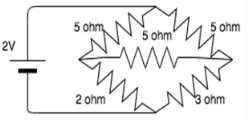Solution:

The 3 5 ohm resistors are connected in delta. Changing it to star:
R1=R2=R3= 1.67 ohm.
One of the 1.67 ohm resistors are connected in series with the 2 ohm resistor and another 1.67 ohm resistor is connected in series to the 3 ohm resistor.
The resulting network has a 1.67 ohm resistor connected in series with the parallel connection of the 3.67 and 4.67 resistors.
The equivalent resistance is: 3.725A.
I=2/3.725= 0.54A.

QUESTION: 9

If a 6 ohm, 2ohm and 4ohm resistor is connected in delta, find the equivalent star connection.

Solution:

Using the delta to star conversion formula:
R1=2*6/(2+6+4)
R2=2*4/(2+6+4)
R3=4*6/(2+6+4).

QUESTION: 10

If a 4ohm, 3ohm and 2ohm resistor is connected in delta, find the equivalent star connection.

Solution:

Using the delta-star conversion formula:
R1=4*3/(2+3+4)
R2=2*3/(2+3+4)
R3=2*4/(2+3+4).

QUESTION: 11

Find the equivalent delta circuit.​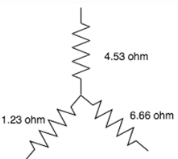Solution:

Using the star to delta conversion:
R1=4.53+6.66+4.53*6.66/1.23
R2=4.53+1.23+4.53*1.23/6.66
R3=1.23+6.66+1.23*6.66/4.56.

QUESTION: 12

Which, among the following is the correct expression for star-delta conversion?

Solution:

After converting to delta, each delta connected resistance is equal to the sum of the two resistance it is connected to+product of the two resistances divided by the remaining resistance. Hence R1=Ra+Rb+Ra*Rb/Rc, R2=Rc+Rb+Rc*Rb/Ra, R3=Ra+Rc+Ra*Rc/Rb.

QUESTION: 13

Find the equivalent resistance between X and Y.​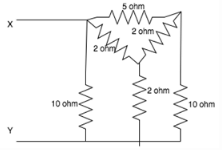Solution:

The two 2ohm and one 5ohm resistors are connected in delta, changing them to star, we have R1=R3= 5*2/(5+2+2) = 10/9ohm,  R2 = 2*2/(9)ohm
The one 10/9ohm and one 4/9ohm resistors are connected in series to the 10 ohm and 2 ohm respectively.
This network can be further reduced to a network consisting of a 11.11ohm and 2.44ohm resistor connected in parallel and then series with 10/9 whose resultant is intern connected in parallel to the 10 ohm resistor.

QUESTION: 14

Delta connection is also known as____________

Solution:

Delta connection is also known as mean connection because its structure is like a mesh, that is, a closed loop.

QUESTION: 15

Rab is the resistance between the terminals A and B, Rbc between B and C and Rca between C and A. These 3 resistors are connected in star connection. After transforming to delta, the resistance at A will be?

Solution:

After converting to delta, each delta connected resistance is equal to the sum of the two resistances it is connected to+product of the two resistances divided by the remaining resistance. Hence, resistance at A= Ra+Rb+Rc*Rb/Rc.

QUESTION: 16

Rab is the resistance between the terminals A and B, Rbc between B and C and Rca between C and A. These 3 resistors are connected in star connection. After transforming to delta, the resistance at B will be?

Solution:

After converting to delta, each delta connected resistance is equal to the sum of the two resistances it is connected to+product of the two resistances divided by the remaining resistance. Hence, resistance at B= Ra+Rc+Ra*Rc/Rb.

QUESTION: 17

Rab is the resistance between the terminals A and B, Rbc between B and C and Rca between C and A. These 3 resistors are connected in star connection. After transforming to delta, the resistance at C will be?

Solution:

After converting to delta, each delta connected resistance is equal to the sum of the two resistance it is connected to+product of the two resistances divided by the remaining resistance. Hence, resistance at C= Ra+Rb+Ra*Rb/Rc.

QUESTION: 18

Delta connection is also known as____________

Solution:
QUESTION: 19

If a 8/9ohm, 4/3ohm and 2/3ohm resistor is connected in star, find its delta equivalent.

Solution:

Using the formula for star to delta conversion:
R1=8/9+4/3+(8/9)*(4/3)/(2/3)
R2=8/9+2/3+(8/9)*(2/3)/(4/3)
R3=2/3+4/3+(2/3)*(4/3)/(8/9).

QUESTION: 20

Find the equivalent resistance between A and B.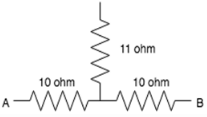Solution:

The equivalent resistance between node 1 and node 3 in the star connected circuit is R=(10×10+10×11+11×10)/11=29ohm.Use Code STAYHOME200 and get INR 200 additional OFF Use Coupon Code